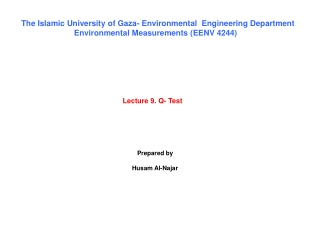DownloadDownload PresentationLecture 9. Q- Test

# Lecture 9. Q- Test

Download Presentation## Lecture 9. Q- Test

- - - - - - - - - - - - - - - - - - - - - - - - - - - E N D - - - - - - - - - - - - - - - - - - - - - - - - - - -
##### Presentation Transcript

1. The Islamic University of Gaza- Environmental Engineering Department Environmental Measurements (EENV 4244) Lecture 9. Q- Test Prepared by Husam Al-Najar

2. The Q test. The Q test uses the range to determine whether a questionable result should be rejected when n= 3 to 10. This test is most commonly conducted at 90% confidence level, which means that the questionable result may be rejected with 90% statistical confidence that it is significantly different from the other results. The rejection quotient is labeled Q0.90; at the 96% confidence level it is designated Q0.96, etc. values for rejection quotients have been compiled by Dixon and are given in Table 1. To perform the Q test, the results are arranged in increasing order of magnitude and labled X1, X2, ……, Xn. The difference between a questionable result and its nearest neighbor is divided by the range to obtain a quotient, Q. If this Q is equal to or greater than the quotient given in Table 1 for n results, the questionable one is rejected.

3. Note: if Q > Q0.90, reject X? . Arrange sample in order of increasing magnitude.

4. Example: An analysis in triplicate gives the following results: 30.13%, 30.20%, and 31.23%. should any of the results be rejected ? Using the Q test, the smallest results is retained. The calculation of the quotient Q for the largest result of 31.23% is as follows : Since Q is equal to Q0.90 (Table 1), the questionable result may be rejected, but just barely. If possible, it would be better to analyze an additional sample than to report the average of the two remaining results.

5. Example: Use the Q test to determine whether any of the following results should be rejected: 40.12, 40.15, 40.55. The close agreement of the first two results makes it tempting to discard the third result. However, the Q test will not quite permit this. ( 0.94 needed for rejection ) Unconvinced by the failure of the Q test to reject a result he “knew” was not as good as the first two, the analyst decided to do two additional analysis. The new results were: 40.20, 40.28. the average of the five results is 40.26, which is much closer to the average of the original three results 40.27 than to the average of the two lower original results (40.14) In this case the additional analyses supported the retention of all of the first three results. Of course, the additional analyses could have been lower and showed that the 40.14 average was more correct. But unless additional analyses are made, it is better statistically to average all three unless one can be rejected by the Q test.

6. When there are more than three results, it may be necessary to test more than one questionable results by the Q test the smallest values is tested first. If it is rejected, the largest value of the remaining set is then tested Example: Use the Q test to test the seven results in this sample: 5.12, 6.82, 6.12, 6.32, 6.22, 6.32, 6.02. Arrange the sample in order of increasing magnitude and label them as shown in Table 2. The smallest result 5.12 is labeled X1 and tested first. Q is calculated as follows:

7. Table 2: Test applied to a sample of seven results Since Q is greater than 0.51 ( Q0.90 for n = 7 ), the smallest result of 5.12 is rejected.

8. As shown in table 2, the values are labled before the largest result, 6.82, is tested. This result retains its lable of Xn. Q is calculated as follows: Since Q is greater than 0.56 ( Q0.90 for n = 6 ), the largest result of 6.82 is rejected. As indicated in table, 6.32 and 6.32 are relabeled as Xn and Xn-1 before 6.02 is tested as the next smallest results. This result retains its label of X1, Q is calculated as follows: Since Q is less than 0.64 ( Q0.90 for n = 5 ), 6.02 is retained. The new largest value of 6.32 should be tested next, but as it is the same as its nearest neighbor, it would be retained by the test.